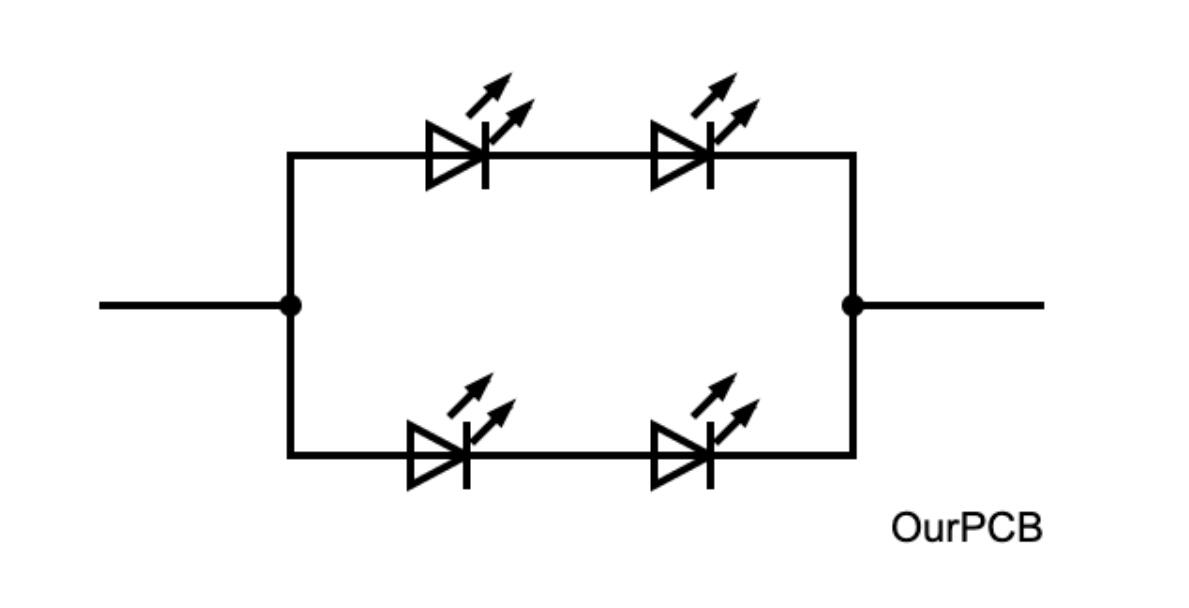Blog  /  Current Limiting Resistor: Protective Resistor for Regulating Current

# Current Limiting Resistor: Protective Resistor for Regulating Current

Resistors are arguably the most typical components used in electrical circuits, and they play a critical role in diode circuits, such as rectifiers and LED strips. These resistors are known as current limiting resistors because of their role in those circuits. We will analyze these electronic components to show how you can calculate their ideal values for your project. Take a look!

## What Is a Current Limiting Resistor?

A current limiting resistor is a resistor connected in series to a circuit for protection against excessive burning in the appliance. It operates on the principle of reducing current by increasing the overall load resistance.A current limiting resistor and a variable resistor in a laser diode circuit diagram

Most electronic components have a limit on the maximum current they can handle. If you exceed this current limit, the parts will not work and might burn out. So any resistor in series with the circuit regulates the current moving through, and you can refer to it as a current limiter resistor.

The device is typical in light-emitting diode circuits to protect them from burning out.

## Why Current Limiting Resistors Are Necessary for LED Light Circuits

LED lights are some of the most fundamental output devices in open-source projects because they provide feedback on a circuit's status in an easy-to-understand manner. For instance, they can show when a device is on. However, connecting an LED directly to a power source will burn it out.

Here's why.

Resistors behave according to ohm's law (V=IR), which is linear. So if the resistance value remains constant, a voltage increase across the resistor will increase the current. And a voltage drop means lower current flow.

But LEDs don't operate like that. They are diodes and behave using the I-V curve. They have a recommended or characteristic forward voltage (usually between 1.5V and 4V) that you must reach to turn on the LEDs.LED circuits with resistors

But once they turn on, the LED resistance drops quickly, and the diodes will allow high current levels to flow across. This excessive current will make them super bright and possibly burn out. A load resistor in series controls the current flowing through and protects the components.

Any circuit with diodes, like a rectifier, requires these series resistors for protection.

The alternative is to use a constant current LED driver, which produces a single current output while varying the voltage. But these drivers are expensive and have limited flexibility. So most people use constant voltage power supplies with current-limiting resistors.An LED driver

## Calculating Current Limiting Resistor Values for LED Circuits

The best way to maximize the life of LEDs is to limit the current flowing through them. Here's how to calculate the correct resistor value for your project.

### Single LEDs

Ohm's law becomes a little complicated when calculating the current limiting resistor value for single LEDs, as shown below.### LEDs in Series

With LEDs connected in series, the formula becomes more complex because the voltage drop across them increases. And it drops the voltage across the resistor, as well. But the current across the resistor remains the same.LEDs connected in seriesWhere n is the number of LEDs in the series.

### LEDs in Parallel

When you connect LEDs in parallel, the current across the resistor increases, but the current flowing through each LED remains constant. On the other hand, the voltage drop through the resistor and LEDs remains unaffected.LEDs connected in series and parallelBut it is vital to have n series LEDs in every parallel branch (m), and the units should have the same forward voltage and current. Otherwise, the formula will be irrelevant.

For instance, consider these four LEDs.LEDs arranged in an array.LEDs are arranged in an array.

The equation does not apply to the first circuit diagram but works for the second.

### Current Limiting Resistor: Controlling Brightness

Some LED applications require dimming functions, such as in ambient lighting. This function requires two resistors.

• A fixed load resistor for limiting current (Rf)
• A variable resistor for brightness adjustment (Rv)A variable resistor

Rf limits current when Rv is at its minimum setting (0Ω) because maximum current flows across. So you can calculate the value of Rf using the following formula when Rv=0.Adjusting Rv increases resistance in the circuit, lowering the current flowing across the LED. So it is lowest when Rv is at the maximum setting.

You can calculate the value of Rv using the following formula.## Current Limiting Resistor: Circuit Design Steps

Use the following four steps to select the proper resistor value for limiting current.

• Use the desired LED specs and operating characteristics in the equations above to get the resistor values.
• Round off the ideal resistor value to get the real value. For instance, if the calculation gives you 133.42Ω, the real value will be 130Ω or 150Ω with a 5% tolerance. But you can select other values based on the specific application or what you have.The meaning of the color coding used in resistors. Note the tolerance color coding.

• Replace the ideal values with the real values in the equations to see if they meet the required operating characteristics.
• Run these calculations using the values at extreme tolerances. The 150Ω resistor will not be as accurate as the 130Ω type because its 5% tolerance is 7.5Ω. So its range will be 142.5Ω to 157.5Ω. Remember to calculate the resistor power dissipation and circuit current draw.

## Wrap up

In conclusion, current limiting resistors are essential for electronic circuits with diodes, like LEDs, due to their I-V curve. And you can calculate the resistor value using the formulas above depending on your LED arrangement. That's it for now. If you have any sentiments or questions, leave a comment, and we'll be in touch.